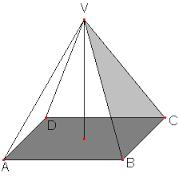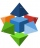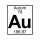# Metal pyramid

Find the weight of a regular quadrilateral pyramid with a 5 cm length and 6,5 cm body height is made from material with density g/cm3.

m =  433.3 g

### Step-by-step explanation:We will be pleased if You send us any improvements to this math problem. Thank you!Tips to related online calculators
Tip: Our volume units converter will help you with the conversion of volume units.
Tip: Our Density units converter will help you with the conversion of density units.

## Related math problems and questions:

• Regular quadrilateral pyramidFind the volume and surface of a regular quadrilateral pyramid if the bottom edge is 45 cm long and the pyramid height is 7 cm.
• Special bodyAbove each wall of a cube with an edge a = 30 cm, a regular quadrilateral pyramid with a height of 15 cm is constructed. Find the volume of the resulting body.
• The prison ballCalculate the density of the material that the prison ball is made from if you know its diameter is 15cm and its weight is approximately 2.3kg. With the help of mathematical-physicochemical tables estimate what material the ball is made from.
• Metal tubeCalculate the metal tube mass 8dm long with the outer radius 5cm and the inner radius 4.5cm and 1cm3 of this metal is 9.5g.
• Quadrilateral pyramidIn a regular quadrilateral pyramid, the height is 6.5 cm and the angle between the base and the side wall is 42°. Calculate the surface area and volume of the body. Round calculations to 1 decimal place.
• Cuboid 5Calculate the mass of the cuboid with dimensions of 12 cm; 0.8 dm and 100 mm made from spruce wood (density = 550 kg/m3).
• Quadrilateral pyramidWe have a regular quadrilateral pyramid with a base edge a = 10 cm and a height v = 7 cm. Calculate 1/base content 2/casing content 3/pyramid surface 4/volume of the pyramid
• Pyramid heightFind the volume of a regular triangular pyramid with edge length a = 12cm and pyramid height h = 20cm.
• Unknown metalThe prism made of unknown metal has dimensions of 3 cm by 3 cm by 5 cm and a weight of 121 g. What metal is it? Write its chemical symbol.
• Quadrilateral pyramidCalculate the volume of a quadrilateral pyramid, the base of which has the shape of a rectangle with dimensions a = 6cm, b = 4cm and height v = 11cm
• Find theFind the surface area of a regular quadrilateral pyramid which has a volume of 24 dm3 and a height of 45 cm.
• The quadrilateral pyramidThe quadrilateral pyramid has a rectangular base of 24 cm x 3.2dm and a body height of 0.4m. Calculate its volume and surface area.
• The regularThe regular quadrilateral pyramid has a volume of 24 dm3 and a height of 45 cm. Calculate its surface.
• Quadrilateral pyramidFind the height and surface of a regular quadrilateral pyramid with a base edge a = 8cm and a wall height w = 10cm. Sketch a picture.
• Triangular pyramidCalculate the volume of a regular triangular pyramid with edge length a = 12cm and pyramid height v = 20cm.
• Surface of pyramidIn a regular quadrilateral pyramid, the height of the sidewall is equal to the length of the edge of the base. The content of the sidewall is 32 cm2. What is the surface of the pyramid?
• Copper plateCalculate the thickness of the copper plate with a density 8.7 g/cm³ measuring 1.5 meters and 80 cm and its weight is 3.65 kg